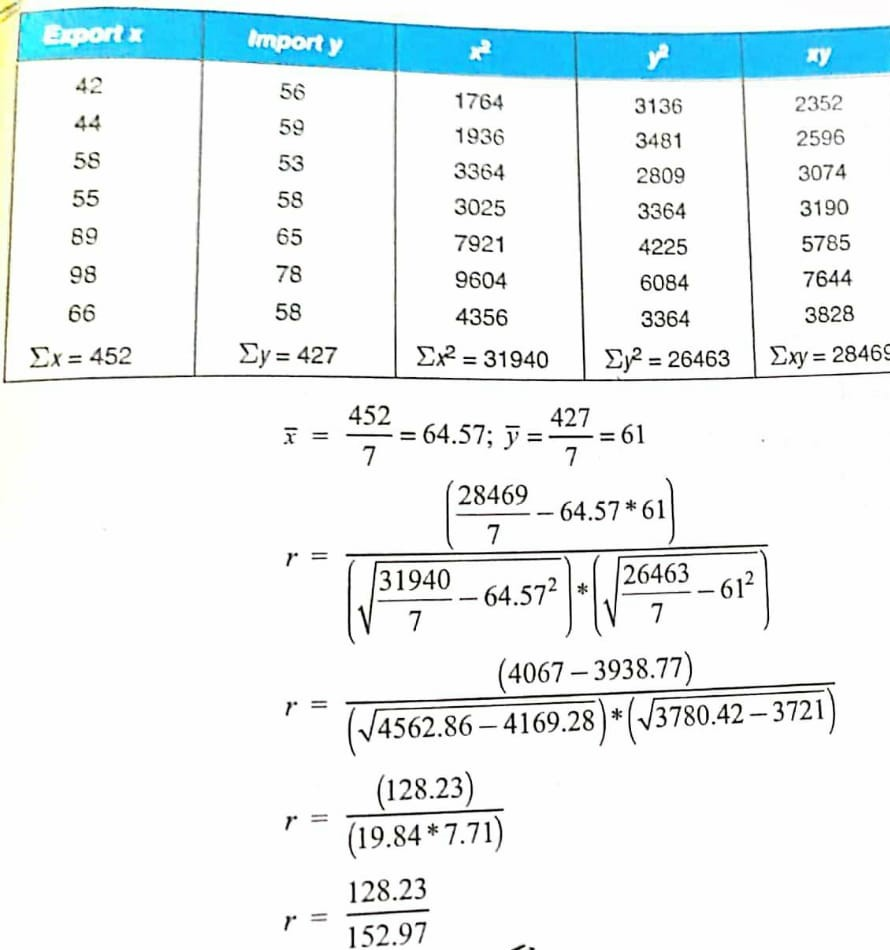# Correlation and Regression : Caiib Paper 1 (Module B), Unit 3

## Correlation and Regression : Caiib Paper 1 (Module B), Unit 3

Dear Bankers,
We all know that CAIIB exams are conducted by the Indian Institute of Banking and Finance (IIBF).  CAIIB is said to be one of the difficult courses to be cleared for the bankers. But we assure you that with the help of our “CAIIB study material”, you will definitely clear the CAIIB exam.
CAIIB exams are conducted twice in a year. Candidates should have completed JAIIB before appearing for CAIIB Exam. Here, we will provide detailed notes of every unit of the CAIIB Exam on the latest pattern of IIBF.
So, here we are providing “Unit 3: Correlation and Regression of “Module B: Business Mathematics” from “Paper 1: Advanced Bank Management (ABM)”.

The Article is Caiib Unit 3: Correlation and Regression

#### ♦Correlation Analysis

• Correlation analysis is applied in quantifying the association between two continuous variables, for example, an dependent and independent variable or among two independent variables.

#### ♦Regression Analysis

##### Linear Regression
• Linear regression is a linear approach to modelling the relationship between the scalar components and one or more independent variables. If the regression has one independent variable, then it is known as a simple linear regression. If it has more than one independent variables, then it is known as multiple linear regression. Linear regression only focuses on the conditional probability distribution of the given values rather than the joint probability distribution. In general, all the real world regressions models involve multiple predictors. So, the term linear regression often describes multivariate linear regression.
##### Correlation and Regression DifferencesThere are some differences between Correlation and regression.

• Correlation shows the quantity of the degree to which two variables are associated. It does not fix a line through the data points. You compute a correlation that shows how much one variable changes when the other remains constant. When r is 0.0, the relationship does not exist. When r is positive, one variable goes high as the other goes up. When r is negative, one variable goes high as the other goes down.
• Linear regression finds the best line that predicts y from x, but Correlation does not fit a line.
• Correlation is used when you measure both variables, while linear regression is mostly applied when x is a variable that is manipulated.
##### Comparison Between Correlation and Regression
 Basis Correlation Regression Meaning A statistical measure that defines co-relationship or association of two variables. Describes how an independent variable is associated with the dependent variable. Dependent and Independent variables No difference Both variables are different. Usage To describe a linear relationship between two variables. To fit the best line and estimate one variable based on another variable. Objective To find a value expressing the relationship between variables. To estimate values of a random variable based on the values of a fixed variable.

Correlation and Regression Statistics

• The degree of association is measured by “r” after its originator and a measure of linear association. Other complicated measures are used if a curved line is needed to represent the relationship.The above graph represents the correlation.

The coefficient of correlation is measured on a scale that varies from +1 to -1 through 0. The complete correlation among two variables is represented by either +1 or -1. The correlation is positive when one variable increases and so does the other; while it is negative when one decreases as the other increases. The absence of correlation is described by 0.

#### Correlation Coefficient Formula#### Simple Linear Regression Equation

As we know, linear regression is used to model the relationship between two variable. Thus, a simple linear regression equation can be written as:
Y = a + bX
Where,

Y = Dependent variable

X = Independent variable

a = [(∑y)(∑x2) – (∑x)(∑xy)]/ [n(∑x2) – (∑x)2]

b = [n(∑xy) – (∑x)(∑y)]/ [n(∑x2) – (∑x)2]

#### Read More Article Caiib Paper-1, Module A

Visit Online Mock test Site: test.ambitiousbaba.com